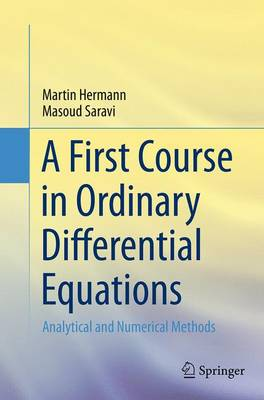•# A First Course in Ordinary Differential Equations: Analytical and Numerical Methods (Paperback)

(author), (author)
£44.99
Paperback 288 Pages / Published: 03/09/2016
• Not available

This product is currently unavailable.

This book presents a modern introduction to analytical and numerical techniques for solving ordinary differential equations (ODEs). Contrary to the traditional format-the theorem-and-proof format-the book is focusing on analytical and numerical methods. The book supplies a variety of problems and examples, ranging from the elementary to the advanced level, to introduce and study the mathematics of ODEs. The analytical part of the book deals with solution techniques for scalar first-order and second-order linear ODEs, and systems of linear ODEs-with a special focus on the Laplace transform, operator techniques and power series solutions. In the numerical part, theoretical and practical aspects of Runge-Kutta methods for solving initial-value problems and shooting methods for linear two-point boundary-value problems are considered.
The book is intended as a primary text for courses on the theory of ODEs and numerical treatment of ODEs for advanced undergraduate and early graduate students. It is assumed that the reader has a basic grasp of elementary calculus, in particular methods of integration, and of numerical analysis. Physicists, chemists, biologists, computer scientists and engineers whose work involves solving ODEs will also find the book useful as a reference work and tool for independent study. The book has been prepared within the framework of a German-Iranian research project on mathematical methods for ODEs, which was started in early 2012.

Publisher: Springer, India, Private Ltd
ISBN: 9788132235279
Number of pages: 288
Weight: 4628 g
Dimensions: 235 x 155 x 16 mm
Edition: Softcover reprint of the original 1st ed. 201